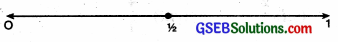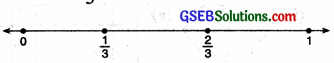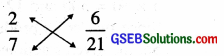# GSEB Class 6 Maths Notes Chapter 7 Fractions

This GSEB Class 6 Maths Notes Chapter 7 Fractions covers all the important topics and concepts as mentioned in the chapter.

## Fractions Class 6 GSEB Notes

Introduction:
In previous classes, we have studied that if an object is divided in equal parts then one or more parts of the object is called fraction. A fraction means a part of whole or a group.
Fraction = $$\frac{\text { Parts of an object }}{\text { Total part of an object }}$$
The upper part of a fraction is called numerator and the lower part is called denominator.

Fractional Part of a Collection:
Fraction:
Fraction is a number representing part of a whole. The whole may be a single object or a group of objects.
A fraction has two parts – the numeral above the division line and the numeral below the division line.

• The numeral below the division line tells into how many equal parts each whole is divided. It is called the denomenator.
• The numeral above the division line tells how many of the parts have been taken. It is called the numerator.Representing a fraction on a number line:
Suppose we want to represent the fraction $$\frac{1}{2}$$ on the number line. We know $$\frac{1}{2}$$ lies between 0 and 1. Take a point dividing the distance between 0 and 1 into two equal parts.

This point represents the fraction $$\frac{1}{2}$$Similarly taking two points which divide the distance between 0 and 1 in three equal parts.
The first point represents second $$\frac{1}{3}$$ represents $$\frac{2}{3}$$In the same way other fractions can be represented.

Types of Fraction
Proper fraction:
If the numerator of a fraction is less than the denominator the fraction is known as proper fraction. Proper fractions lie between 0 and 1.
For example. $$\frac{2}{3}, \frac{1}{4}, \frac{3}{7}$$

Improper Fraction:
If the numerator of a fraction is greater than the denominator the fraction is known as improper fraction.
For example. $$\frac{5}{2}$$ is an improper fraction and can be written as 2$$\frac{1}{2}$$ In this form it is called a mixed number.

To express an improper fraction to a mixed number, divide the numerator by denominator then
Improper fraction = Quotient $$\frac{\text { Remainder }}{\text { Divisor }}$$

We can also express mixed fraction as an improper fraction by multiplying the whole number with denominator and adding numerator to it. Then,
Improper fraction = $$\frac{(\text { Whole number } \times \text { Denominator })+\text { Numerator }}{\text { Denominator }}$$

Equivalent Fractions

• An equivalent fraction of a given fraction can be obtained by multiplying its numerator and denominator by the same number (other than 0)
• An equivalent fraction of a given fraction can be obtained by dividing its numerator and denominator by their common factor (other than 1) if any.
• If two fractions are equivalent, the product of numerator of the first and denominator of the second is equal to the product of the denominator of the first and numerator of second.

The simplest form of Fractions
A fraction is said to be in simplest and lowest form if its numerator and denominator have no common factor except 1.

Checking for Equivalent Fractions (by Cross product)
If the product (multiplication) of the numerator of the first and the denominator of the second is equal to the product of the denominator of the first and the numerator of the second than the given fractions are said to be equivalent consider an example.2 × 21 = 42 and 6 × 7 = 42 Both the cross products are same.
Thus two fractions are equivalent if their cross products are equal.

Like, Unlike and Unit Fractions:
Like Fractions:
Fractions with same denominator are called like fractions.
For Example, $$\frac{1}{13}, \frac{3}{13}, \frac{8}{13}, \frac{10}{13}$$ are fractions.

Unlike Fractions:
Fractions with different numerator are called unlike fractions.
For Example. $$\frac{1}{7}, \frac{2}{13}, \frac{3}{10}$$ are all unlike fractions.

Unit Fractions: A fraction having 1 as its numerator is called unit fraction.
For Example. $$\frac{1}{2}, \frac{1}{5}, \frac{1}{7}, \frac{1}{8}$$ are all unit 2 5 7 8 fractions.

Comparing and Ordering of Fractions
Comparing like Fractions.

• Of the two fractions having the same denominator the one with greater numerator is greater.
• Of the two fraction having the same numerator, the one with greater denominator is smaller.

Fraction with different numerators and denominators
To compare the fractions with different numerators and denominators. First we convert that fractions into like fractions using the following steps :

• Step 1. Find the L.C.M. of the denominators of the fractions.
• Step 2. Convert each fraction into its equivalent fraction with denominator equal to the L.C.M. obtained in Step 1.
• Step 3. Compare the numerators of the equivalent fractions whose de-nominator are same.Operations on Fractions
Addition and Subtraction of like fractions. Like fractions can be added by simply adding their numerator and taking the same denominator.
For Example.
$$\frac{2}{7}+\frac{4}{7}+\frac{6}{7}=\frac{2+4+6}{7}=\frac{12}{7}$$

Like fractions can also be substracted by simply subtracting their numerator and taking same denominator.
For Example.
$$\frac{6}{7}-\frac{5}{7}=\frac{6-5}{7}=\frac{1}{7}$$

Thus, the sum and difference of two or more like fractions can be obtained as follows:

• Step 1. Add/Subtract the numerator of all fractions.
• Step 2. Retain the common denominator of all fractions.
• Step 3. Write the fraction as $$\frac{\text { Sum/difference of numerators }}{\text { Common denominator }}$$

Addition and Subtraction of Unlike fractions.
First we convert unlike fractions to equivalent like fractions and then we add or subtract, we follow these steps :

• Step 1. Find the L.C.M. of the denominators.
• Step 2. Convert each fraction into an equivalent fraction with denominator equal to the L.C.M. obtained in step 1.
• Step 3. Add or subtract like fractions as